# Quantum Mechanical Model of the Atom Chemistry Tutorial

## Key Concepts

• Louis de Broglie proposed a model for the atom based on the wave properties of electron "particles"
Bohr's "orbits" were replaced with de Broglie's "standing waves".

Only whole numbers of wavelengths are allowed.

• Equivalent quantum mechanical models of the atom were developed independently by Werner Heisenberg and Erwin Schrödinger.
Schrödinger's quantum mechanical model was preferred because some of its ideas could be visually represented.
• Quantum mechanical model of the atom is based on the probability of finding an electron within a volume of space around the nucleus.
• The probability of finding an electron within a volume of space is dependent on the distance from the nucleus, there are volumes in which it is highly likely an electron will be found, and volumes in which it is very unlikely an electron will be found.
• This "distribution" of probability is referred to as "probability density".

Chemists often use the term "charge cloud" instead of probability density.

• The term "orbital" refers to the shape of the volume of space in which there is the greatest probability density.
• In general:
(a) Energy levels (shells) contain energy sub-levels (subshells).

(b) Energy sub-levels (subshells) contain a number of orbitals.

No ads = no money for us = no free stuff for you!

## de Broglie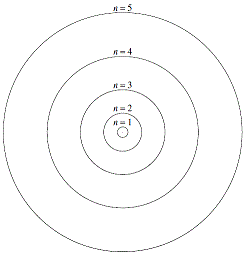The Bohr model for the atom, proposed by Niels Bohr in 1913, had negatively charged particles called electrons orbiting around the positively charged nucleus in fixed circular orbits as shown on the right, much like the planets orbit the sun.1
Orbits close to the nucleus were of less energy than orbits further away from the nucleus.
This model was designed to explain the hydrogen emission spectrum, which it did quite well, but it was an uncomfortable mix of classical physics and the quantisation of energy.
It did not answer the question of why electrons should move in circular orbits at fixed distances from the nucleus.

In 1905, Albert Einstein showed that light could be considered as both a wave and a particle. This is referred to as the wave-particle dualism of radiation.
In 1924, French physicist Louis de Broglie suggested that particles might also have a wave-particle dualism and sometimes act as waves.
He said that the wavelength (λ) of a particle should be inversely proportional to both the :

• mass of the particle (m): λ ∝ 1/m
• speed of the particle (ν): λ ∝ 1/ν

Increasing the mass of a particle reduces its wavelength.
Increasing the speed of a particle reduces its wavelength (and thereby increases its frequency)
In the Bohr model of the atom, an electron close to the nucleus has less energy (lower speed) than an electron further from the nucleus (faster moving electron).
If electrons can be considered as a wave, then an electron close to the nucleus would have a longer wavelength than an electron further from the nucleus.

Louis de Broglie realised that if an electron is confined to an orbit around the nucleus, standing waves would be set up, that is, the orbit of an electron would correspond to a fixed whole number of wavelengths for the electron. Fractions of wavelengths are not allowed. You can have 1 wavelength or 2 wavelengths, but you can't have ½ a wavelength nor can you have 1.75 wavelengths.2
Close to the nucleus, n=1 of the Bohr model, the energy of the electron provides for an orbit corresponding to 1 wavelength. The next orbit, n=2, corresponds to an orbit two wavelengths long. Then the n=3 orbit can fit three wavelengths, etc

 Bohr Orbit Numer of allowed wavelengths Representation dotted line = Bohr orbit solid line = wave n = 1 n = 2 n = 3 1 allowed wavelength 2 allowed wavelengths 3 allowed wavelengths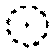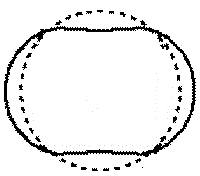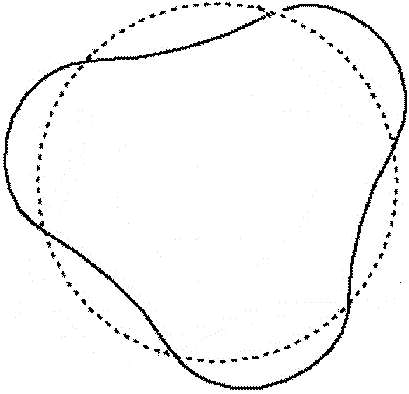de Broglie replaced Bohr's stable "orbits" with "standing wave modes".

Evidence of the wave behaviour of electrons was found in 1927 by American physicists Davisson and Germer who observed electron diffraction, diffraction being a property of waves.3

Louis de Broglie's standing wave model of the atom can be used to successfully describe the hydrogen emission spectrum. Each standing wave is an allowed quantum state.
The ground state, the state before an electron absorbs energy, corresponds to quantum number n=1
An electron can absorb a quanta of energy, leaping to a higher, excited quantum state, n=2, n=3, etc depending on how much energy was absorbed.
Eventually, an electron in an excited state will emit a quanta of energy and leap down to a lower quantum state.

But de Broglie's standing wave model of the atom still cannot explain the different relative intensities of the lines in the hydrogen emission spectrum.

Do you know this?

Play the game now!

## Schrödinger

de Broglie's standing wave model of the atom explained why the energy of electrons was quantised, but it raised a new question.

A wave involves some sort of vibration, for example, in a photon of light there are vibrating electric and magnetic fields. But an electron wave is not a form of electromagnetic radiation, it does not travel at the speed of light and it does have a non-zero rest mass.
So what is vibrating in the electron wave? The best answer is probably "probability"!

In the middle of the 1920s, quantum mechanics or wave mechanics, an abstract mathematical model combining the wave and particle theories, was developed independently by German physicist Werner Heisenberg4 and Austrian physicist Erwin Schrödinger. English physicist Paul A. M. Dirac showed that these two forms of the model were equivalent.5

The theory developed by Schrödinger gained greater acceptance by physicists because some of its ideas could be represented pictorially. Schrödinger's theory produced a differential equation incorporating a wave equation and Planck's constant to permit quantisation of energy.
The solution of the equation involves finding the wave function, Ψ. The wave function is complex and does not describe a real, physical wave.
The square of the absolute value of the wave function, |Ψ|2, however, is real and it describes the probability, or chance, of finding a particle in a given position at a given time.
Since |Ψ|2 is the probability of finding an electron within a volume of space, it is a probability per unit volume, and is therefore called a "probability density".6Solving the Schrödinger equation for the hydrogen atom we find that there is a significant probability of finding the electron at any position within a spherically shaped volume surrounding the nucleus.
The probability of finding the electron close to the nucleus is greater than the probability of finding the electron further away from the nucleus. This probability of finding the electron within a volume of space can be represented 2-dimensionally as shown on the right (just remember that the actual space is a 3-dimensional volume though).Each dot in the diagram represents the probability of finding the electron in this spot. So the probability density is greatest closer to the nucleus than further away from it, in the diagram we see that the density of dots, the number of dots in a given area, is greatest nearer the nucleus at the center of the diagram than it is further away from the nucleus.
Because the electron carries a negative charge, and the chance of finding the electron closer to the nucleus is greater than finding it further away, we can visualise this probability density of charge as being "smeared out" within a spherical volume of space for the hydrogen atom. Chemists often referred to this "smeared out" volume of charge as a "charge cloud".

The electron clearly does NOT travel in a fixed circular orbit around the nucleus as described by Bohr.
Quantum mechanics now tells us we can not determine the precise location of a given electron, we can only refer to volumes of larger and smaller probability density.
The term "orbital" (NOT orbit) is used to describe the volume of space within which there is a large percentage, say 90%, of probability of finding the electron.
When we draw a circle to represent the spherical orbital for the electron of the hydrogen atom, what we are saying is that there is a 90% (say) chance of finding the electron within that spherical volume of space.

Other solutions to the Schrödinger wave equation give shapes for orbitals that are not spherical.
In order to represent these orbitals in 3 dimensions, we need a way to orientate them in space, so we use a set of 3-dimensional cartesian coordinates (the xyz coordinate system) as a reference.
Representations of the shapes of the s, p and d orbitals and their corresponding symbols are shown below7 :

 s orbital shape symbol p orbitals shape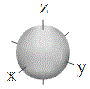s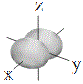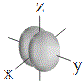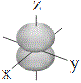px py pz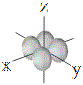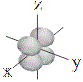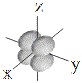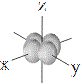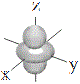dxy dxz dyz dx2-y2 dz2

Solutions to the Schrödinger wave equation provide information about the various energy states for the hydrogen atom, and, can also be used to describe other atoms8 .

First, we can think about the arrangement of electrons around a nucleus in terms of energy levels (also referred to as shells):
n=1 (First energy level or K shell)
n=2 (Second energy level or L shell)
n=3 (Third energy level or M shell)
n=4 (Fourth energy level or N shell)

Every energy level, except n=1, can be split up into a number of energy sub-levels (or subshells) such as the:
s subshell
p subshell
d subshell
f subshell

Every sub-level or subshell, except the s subshells can be split up into a number of orbitals:
3 sets of p orbitals (px, py, pz)
5 sets of d orbitals (dxy, dxz, dyz, dx2-y2,dz2)
7 sets of f orbitals
 ↑↑↑↑ n=2 (L shell) ____ 2p____ 2s____ 2px 2py 2pz___ ___ ___ 2s____ ↑ ↑ ↑↑ n=1 (K shell) ____ 1s____ 1s____ ↑energy levels(shells) sub-levels(subshells) orbitals

Quantum mechanics not only predicts the wavelengths of light in the hydrogen emission spectrum, it also gives us the correct relative intensities of the lines by allowing us to calculate the probability of a particular transition occurring.

Quantum mechanics can even be used to describe the bonding and properties of molecules, but that's another story ...

Do you understand this?

Take the test now!

Footnotes:

1. Sommerfield extended the Bohr model by allowing for elliptical as well as circular orbits, making it possible to semi-quantitatively handle atoms larger than hydrogen. This is referred to as the Bohr-Sommerfield model of the atom.

2. If you use a non-integer multiple of wavelengths, then these waves quickly die out as a result of destructive interference.

3. Electron diffraction proved to be a very useful tool, forming the basis of electron microscopes which enables us to investigate specimens that are smaller than the wavelength of visible light.

4. In 1927 Heisenberg produced a system called matrix mechanics which could be used to give the wavelengths of spectral lines, but did not provide a physical picture of the atom.
Heisenberg is most famous for the formulation of the "uncertainty principle" for which he was awarded the 1932 Nobel Prize in Physics.

5. Dirac was awarded the 1933 Nobel Prize in Physics for his work on wave mechanics and his theory of antiparticles. This Nobel Prize was shared with Schrödinger for his work on wave mechanics.

6. Recall that "density" is defined the mass of substance per unit volume.

7. S, P, D and F states were observed spectroscopically and named after the characteristics of the spectra; S for sharp, P for principal, D for diffuse and F for fundamental.
Later, the same letters, but in lower case, were applied to the orbitals, hence, s, p, d and f.
The next symbol to be used in the series after f would be g.

8. This discussion makes no mention of quantum numbers which can be found in a separate Quantum Numbers Tutorial.